This document contains R versions of the boxed examples from Chapter 13 of the “Analysis and Interpretation of Freshwater Fisheries Data” book. Some sections build on descriptions from previous sections, so each section may not stand completely on its own. More thorough discussions of the following items are available in linked vignettes:

The following additional packages are required to complete all of the examples (with the required functions noted as a comment and also noted in the specific examples below).

> library(FSA)          # fitPlot, hoCoef,lagratio, popSizesPlot, rcumsum, srFuns, srStarts
> library(TTR)          # SMA
> library(popbio)       # eigenanalysis

In addition, external tab-delimited text files are used to hold the data required for each example. These data are loaded into R in each example with read.table(). Before using read.table() the working directory of R must be set to where these files are located on your computer. The working directory for all data files on my computer is set with

> setwd("C:/aaaWork/Web/fishR/BookVignettes/aiffd2007")

In addition, I prefer to not show significance stars for hypothesis test output, reduce the margins on plots, alter the axis label positions, and reduce the axis tick length. In addition, contrasts are set in such a manner as to force R output to match SAS output for linear model summaries. All of these options are set with

> options(width=90,continue=" ",show.signif.stars=FALSE, contrasts=c("contr.sum","contr.poly"))
> par(mar=c(3.5,3.5,1,1),mgp=c(2.1,0.4,0),tcl=-0.2)

## 13.1 Life Table Analysis

Consider the following data obtained for the 1954 cohort of Brook Trout (Salvelinus fontinalis) from Hunt Creek, Michigan (McFadden et al. 1967). Details of sampling methodology and estimation of the life table column data are given in McFadden et al. (1967).

This vignette basically demonstrates how to use R as a calculator. As this is pretty straightforward and the details are found in the box and associated chapter the descriptions here will be reserved for only the more complex calculations.

Throughout this document I use $$m_{x}$$ rather than $$b_{x}$$ to represent the fertility rates. With this change I will use $$k$$ to represent the number of age groups in the analysis.

The results presented here are slightly different from those in the box as R retains many more decimal places than it shows and more decimal places then used in the box. In addition, the iterative procedure for the Euler-Lotka equation near the end of this vignette was solved with a function here but was solved iteratively “by eye” in the box. Thus, the results here will differ slightly from what was presented in the box.

I also found the following errors/typos in this box:

• the subscripts for $$q_{x}$$ and $$p_{x}$$ in the first row of table 13.2 should be $$n_{x}$$. I did not check the rest of the table
• the left-hand-side of equation 13.2 should be “1” (i.e., one) – it appears to be an “l” (i.e. “el”).
• the provided $$S_{x}$$ equation does not seem to be implemented properly in the box. It seems that $$S_{m}$$ should equal 0.5*$$l_{m}$$. In the box it appears to be equal to $$l_{m}$$.
• the $$b_{x}$$ values (note from above that I am calling this $$m_{x}$$) are never fully defined in the chapter or the box (what does “fertility rate” mean exactly?). It appears that the $$b_{x}$$ in the chapter are the number of offspring born to a mother of age $$x$$. If this is true then the top row in the Leslie matrix ($$M$$) on page 579 (equation 13.8) is not correct. The top row should have fecundity ($$f_{x}$$), the per capita average number of female offspring reaching $$n_{0}$$ born from mothers of the age class $$x$$ – i.e., the number of offspring produced at the next age class $$m_{x+1}$$ weighted by the probability of reaching the next age class or $$f_{x}=p_{x}m_{x+1}$$.

### 13.1.2 Preparing Data

The ages (x) in the analysis is entered into a vector below. Note that the colon between two integers results in a vector of sequential integers between the two values. The number of ages (k) in this example is extracted with length() from the number of items in x. The abundance (nx) and fecundity (mx) data were then entered into vectors by combining the values together with c().

> ( x <- 0:4 )
 0 1 2 3 4
> ( k <- length(x) )
 5
> ( nx <- c(52000,2118,779,159,13) )
 52000  2118   779   159    13
> ( mx <- c(0,0,43,122.6,346.2) )
   0.0   0.0  43.0 122.6 346.2

### 13.1.3 Filling Out the Life Table

The vector of number of deaths is computed from the sequential differences in the abundance values. Sequential differences are computed with diff(). However, a zero must be concatenated to the end of the $$n_{x}$$ vector with c() so that the last difference is computed properly. In addition, diff() computes the “second” values minus the “first” values, whereas we want the “first” values minus the “second” values. Thus, the correct number of deaths (dx) is given by multiplying the results from diff() by “-1”. The age-specific mortality rate (qx), age-specific survival rates (px), and the proportion of initial animals that live to age-x (lx) are then computed from the previous results.

> ( dx <- -1*diff(c(nx,0)) )
 49882  1339   620   146    13
> ( qx <- dx/nx )
 0.9592692 0.6322002 0.7958922 0.9182390 1.0000000
> ( px <- 1-qx )
 0.04073077 0.36779981 0.20410783 0.08176101 0.00000000
> ( lx <- nx/nx )
 1.000000000 0.040730769 0.014980769 0.003057692 0.000250000

The average number of animals alive in interval $$x$$ (Lx) can be computed with a simple moving average of adjacent abundance values. The SMA() function from the TDD package computes a simple moving average of adjacent abundance values. This function requires the vector of values as the first argument and the number of adjacent values to compute the moving average in the n= argument. In this case, a “0” must again be added to the vector so that the last average is computed properly. However, the result will be returned with an “NA” in the last position which should then be ignored (which is accomplished by appending [-1]) to the vector.

> ( Lx <- SMA(c(nx,0),n=2)[-1] )
 27059.0  1448.5   469.0    86.0     6.5

Three intermediate calculations are computed below. Two of the functions use rcumsum() (from the FSA package) which computes the reverse cumulative sum – i.e., the sum of the current value and each value after it in the vector.

> ( Tx <- rcumsum(Lx) )
 29069.0  2010.0   561.5    92.5     6.5
> ( ex <- Tx/nx )
 0.5590192 0.9490085 0.7207959 0.5817610 0.5000000
> # rcumsum includes the full last value, subtract half to effectively have added half
> ( Sx <- rcumsum(lx)-0.5*lx[k] )
 1.058894231 0.058894231 0.018163462 0.003182692 0.000125000

The following creates a vector of accidental deaths (ax; a vector of all zeroes as their were no accidental deaths). The second line simply calculates the formula for an intermediate calculation.

> ax <- rep(0,k)
> # last entry below should be NA
> ( Wx <- c((qx[1:(k-1)]*Sx[2:k]^2)/(px[1:(k-1)]*(nx[1:(k-1)]-0.5*ax[1:(k-1)])),NA) )
 1.570942e-06 2.677407e-07 5.070449e-08 1.103653e-09           NA

Put all of the results together into an expanded life table to see what has been accomplished.

> cbind(x,nx,dx,lx,qx,px,mx,Lx,Tx,Sx,Wx,ex)  # for display only
     x    nx    dx          lx        qx         px    mx      Lx      Tx
[1,] 0 52000 49882 1.000000000 0.9592692 0.04073077   0.0 27059.0 29069.0
[2,] 1  2118  1339 0.040730769 0.6322002 0.36779981   0.0  1448.5  2010.0
[3,] 2   779   620 0.014980769 0.7958922 0.20410783  43.0   469.0   561.5
[4,] 3   159   146 0.003057692 0.9182390 0.08176101 122.6    86.0    92.5
[5,] 4    13    13 0.000250000 1.0000000 0.00000000 346.2     6.5     6.5
Sx           Wx        ex
[1,] 1.058894231 1.570942e-06 0.5590192
[2,] 0.058894231 2.677407e-07 0.9490085
[3,] 0.018163462 5.070449e-08 0.7207959
[4,] 0.003182692 1.103653e-09 0.5817610
[5,] 0.000125000           NA 0.5000000

### 13.1.4 Basic Calculations I

The gross reproductive rate (GRR), net reproductive rate (R0), and mean generation time (G) are computed in the first three lines below following calculations shown in the box. The variance and confidence interval for mean life expectancy at birth ($$e_{0}$$) is then computed.

> ( GRR <- sum(mx) )
 511.8
> ( R0 <- sum(lx*mx) )
 1.105596
> ( G <- sum(x*lx*mx)/R0 )
 2.495636
> ( var.e0 <- sum(Wx,na.rm=TRUE) )
 1.890491e-06
> ( CI.e0 <- ex+c(-1,1)*qt(0.975,nx-1)*sqrt(var.e0) )
 0.5563243 0.5617141

### 13.1.5 Solving Euler-Lotka Equation

The function below computes the Euler-Lotka equation sum minus 1 given a value of $$r$$, ages (in $$x$$), $$l_{x}$$, and $$m_{x}$$. Note that the minus 1 means that, with this function, we will be looking for a value of $$r$$ that results in a value close to 0 rather than 1. This modification allows one to use a root finding function to find the value of $$r$$ that provides a value of this function close to zero (within a level of precision). The uniroot() function can be used to find this root. This function requires the function as the first argument and a range of values for the first argument to the function (i.e., $$r$$ in this case) as the second argument, and data vectors for the other arguments to the function as the remaining arguments. The second line below computes an initial value of $$r$$ as shown in the box, which I then used to define a range of values of $$r$$ to supply to the second argument of uniroot(). Appending the $root suffix to the result provides just the value of $$r$$ that results in an Euler-Lotka value of 0. > EL <- function(r,x,lx,mx) sum(exp(-r*x)*lx*mx)-1 > ( r.init <- log(R0)/G )  0.0402241 > ( r.final <- uniroot(EL,c(0.03,0.05),x,lx,mx)$root )
 0.04035567

The following plots (right is a zoomed in version of the plot on the left) show the Euler-Lotka sum against the values of $$r$$ to illustrate the result from above.

> rs <- seq(0.03,0.05,0.00001)
> ELs <- sapply(rs,EL,x,lx,mx)
> plot(ELs~rs,type="l",xlab="Possible r",ylab="Euler-Lotka Value")
> abline(h=0,lty=3)
> abline(v=r.final,lwd=2,lty=3,col="red")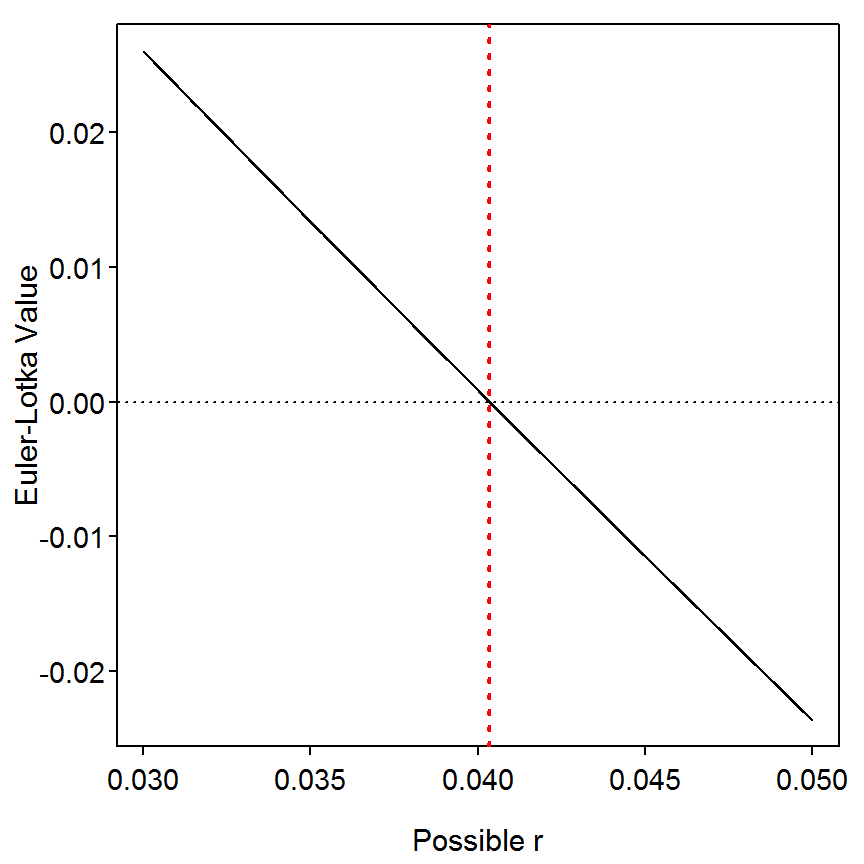> plot(ELs~rs,type="l",xlab="Possible r",ylab="Euler-Lotka Value",xlim=c(0.039,0.041))
> abline(h=0,lty=3)
> abline(v=r.final,lwd=2,lty=3,col="red")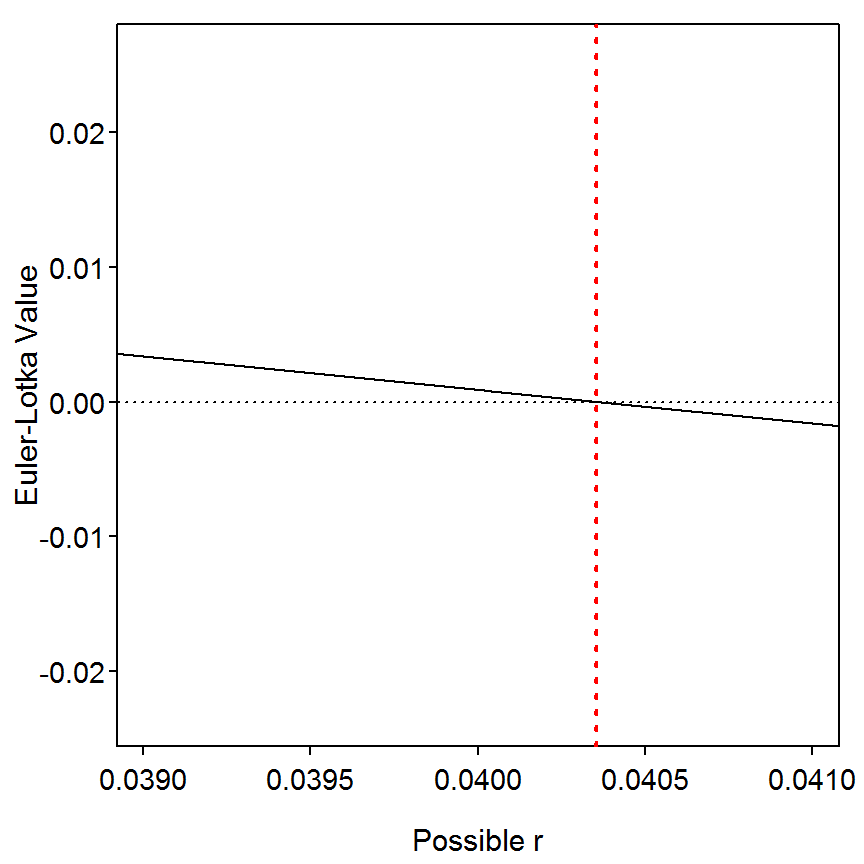### 13.1.6 Basic Calculations II

The finite population rate (lambda), doubling time (DT), and stable age distribution proportions (Cx) are computed as shown below.

> ( lambda <- exp(r.final) )
 1.041181
> ( DT <- log(2)/r.final )
 17.17596
> Cx.int <- lambda^(-x)*lx
> ( Cx <- Cx.int/sum(Cx.int) )
 0.9470946246 0.0370501304 0.0130880516 0.0025657151 0.0002014784

### 13.1.7 Converting Life Table to Leslie Matrix

The $$l_{x}$$ and $$m_{x}$$ portions of the life table can be converted to a Leslie matrix (see Box 13.2 for more information about Leslie matrices). The dominant eigenvalue of the Leslie matrix is an estimate of $$\lambda$$ and the corresponding eigenvector, rescaled to sum to 1, is the stable age distribution. The following function does this conversion (ULTIMATELY I SHOULD DESCRIBE WHAT THIS DOES AS IT IS DIFFERENT FROM EQUATION 13.8).

> lt2lm <- function(x,lx,mx) {
k <- length(x)
px <- c(lagratio(lx),0)
fx <- px[1:(k-1)]*mx[2:k]
M <- cbind(rbind(fx,diag(px[-k],nrow=(k-1))),rep(0,k))
rownames(M) <- colnames(M) <- x
M
}

The Leslie matrix (M) is then constructed in the first line below and eigen.analysis() (from the popbio package) provides the lambda and stable age distribution values from the Leslie matrix.

> ( M <- lt2lm(x,lx,mx) )
           0          1          2           3 4
0 0.00000000 15.8153919 25.0236200 28.30566038 0
1 0.04073077  0.0000000  0.0000000  0.00000000 0
2 0.00000000  0.3677998  0.0000000  0.00000000 0
3 0.00000000  0.0000000  0.2041078  0.00000000 0
4 0.00000000  0.0000000  0.0000000  0.08176101 0
> M.eig <- eigen.analysis(M)
> M.eig$lambda1  1.041181 > M.eig$stable.stage
           0            1            2            3            4
0.9470946266 0.0370501294 0.0130880509 0.0025657148 0.0002014783 
> log(M.eig$lambda1) #shows this lambda is similar to r from above  0.0403557 ## 13.2 Leslie Matrix Model Analysis Consider a simple two-age-class population (age-0 and age-1) with age-class abundances as follows: $$N_{0}=10$$ and $$N_{1}=5$$. Age-specific fecundities are $$b_{0}=10$$ and $$b_{1}=25$$ and age-specific mortality rates expressed as survival probabilities are $$P_{0}=0.5$$ and $$P_{1}=0$$. ### 13.2.1 Preparing Data The “only” data that needs to be entered is the Leslie matrix and a vector of initial populations size for each age. Matrices are entered into R with matrix() which requires a vector of values as the first argument, the number of rows in the nrow= argument, and the fact that values should be entered into the matrix by rows and then columns with the byrow=TRUE argument. The “more complex” Leslie matrix in the box is entered into R as shown below. The columns and rows are then named with colnames() and rownames(), respectively. > M <- matrix(c(0,5,10,0.5,0,0,0,0.2,0),nrow=3,byrow=TRUE) > colnames(M) <- rownames(M) <- 1:3 # 1,2,3 for ages 1-3 > M  1 2 3 1 0.0 5.0 10 2 0.5 0.0 0 3 0.0 0.2 0 The initial population sizes is entered into a vector with c() and the cells named with names()) as shown below. > n0 <- c(0,0,10) > names(n0) <- 1:3 # not really needed, but may help > n0  1 2 3 0 0 10  ### 13.2.2 Population Projections (By Brute Force) The initial population can be projected one step into the future with matrix multiplication as shown in the box. Two matrices or vectors are multiplied in R by placing the %*% operator between the matrix or vector objects. For example, the matrix multiplication $$n_{1}=Mn_{0}$$ is accomplished with the first line below. The second line shows the next step into the future. > ( n1 <- M %*% n0 )  [,1] 1 100 2 0 3 0 > ( n2 <- M %*% n1 )  [,1] 1 0 2 50 3 0 We may iterate the next five steps in the process with a loop as follows (note that cbind() binds items together column-wise). > exp0 <- cbind(n0,n1,n2) # first three values > for (i in 3:7) exp0 <- cbind(exp0,M %*% exp0[,i]) # loop through next five > colnames(exp0) <- 1:8 # name for time steps > exp0 # take a look  1 2 3 4 5 6 7 8 1 0 100 0 250 100 625 500.0 1662.5 2 0 0 50 0 125 50 312.5 250.0 3 10 0 0 10 0 25 10.0 62.5 The total population size at each time step is obtained by summing each column in exp0. This is most easily accomplished with apply() which requires the two-dimensional matrix as the first argument, the margin to which a function will be applied as the second argument (margin=1 for rows and margin=2 for columns), and the function to be applied (i.e., sum()) as the third argument. > ( N_exp0 <- apply(exp0,2,sum) )  1 2 3 4 5 6 7 8 10.0 100.0 50.0 260.0 225.0 700.0 822.5 1975.0  The average finite rate of change in the population total ($$\lambda$$) is computed as the dominant eigenvalue of the Leslie matrix as discussed in the box. The proportional stable age distribution is found by rescaling the eigenvector that corresponds to the dominant eigenvalue to a sum of 1. The eigensystem analysis can be conducted in R with eigen() which requires the Leslie matrix as the first and only argument. The dominant eigenvalue, or estimate of $$\lambda$$, is found by appending $values to the saved eigen object. The dominant eigenvector is found by appending $vectors to the saved eigen object. Dividing this vector by sum of itself produces the stable age distribution proportions. > M.eig <- eigen(M) > M.eig$values
 1.752332
> M.eig$vectors[,1]/sum(M.eig$vectors[,1])
 0.75878278 0.21650655 0.02471067

### 13.2.3 Population Projections (The Easier Way)

The popbio package provides several functions that make the Leslie matrix analysis easier (a paper describing the functions in the popbio package can be obtained here). These functions will be illustrated here using the Leslie projection matrix M and initial population sizes n0 from above. None of the rest of the analysis from the previous section is required for this section.

The initial population can be projected into the future according the Leslie matrix with pop.projection(). This function requires the Leslie matrix as the first argument, the initial population size as the second argument, and the number of iterations into the future as the third argument. For example, the first eight iterations are constructed and saved to an object in the first line below. Various results can be extracted from the saved pop.projection object (the popbio package uses the more generic “stage” rather than “age” as the methods here can be used for transitions between any types of life stages (e.g., YOY, juvenile, adult), not just ages. See section 13.2.2.3 in the AIFFD book. Throughout this document “stage” means “age.”). For example, the

> exp1 <- pop.projection(M,n0,8)
> exp1$stage.vectors  0 1 2 3 4 5 6 7 1 0 100 0 250 100 625 500.0 1662.5 2 0 0 50 0 125 50 312.5 250.0 3 10 0 0 10 0 25 10.0 62.5 > exp1$lambda
 2.401216
> exp1$stable.stage  1 2 3 0.84177215 0.12658228 0.03164557  However, if the user did not want to project the population first, these results could be obtained directly from the Leslie matrix with eigen.analysis(). > M.eig1 <- eigen.analysis(M) > M.eig1$lambda1
 1.752332
> M.eig1$stable.stage  1 2 3 0.75878278 0.21650655 0.02471067  The stable age distribution, generation time, and net reproductive rate can be computed directly from the Leslie matrix using generation.time() and net.reproductive.rate(). > stable.stage(M)  1 2 3 0.75878278 0.21650655 0.02471067  > generation.time(M)  2.233298 > net.reproductive.rate(M)  3.5 The elasticities mentioned in the chapter can be found with elasticity() and visually displayed from the saved object with image2(). These results show that the finite rate of change is strongly related to age-1 survival and age-2 fecundity. > ( M.elast <- elasticity(M) )  1 2 3 1 0.000000 0.37246699 0.08502201 2 0.457489 0.00000000 0.00000000 3 0.000000 0.08502201 0.00000000 > image2(M.elast)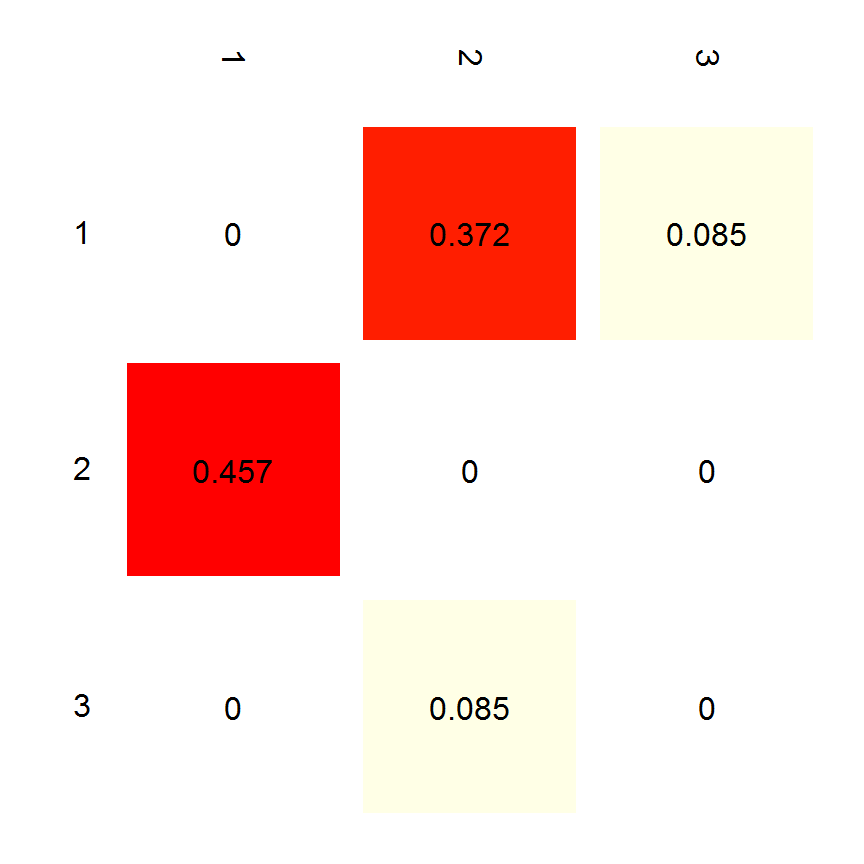Finally, the population can be projected for a few more years to get more interesting results. > exp1 <- pop.projection(M,n0,30) > exp1$lambda
 1.753385
> exp1$stable.stage  1 2 3 0.75896719 0.21630669 0.02472612  A plot of the overall population size is obtained with popSizesPlot(), from the FSA package. > popSizesPlot(exp1)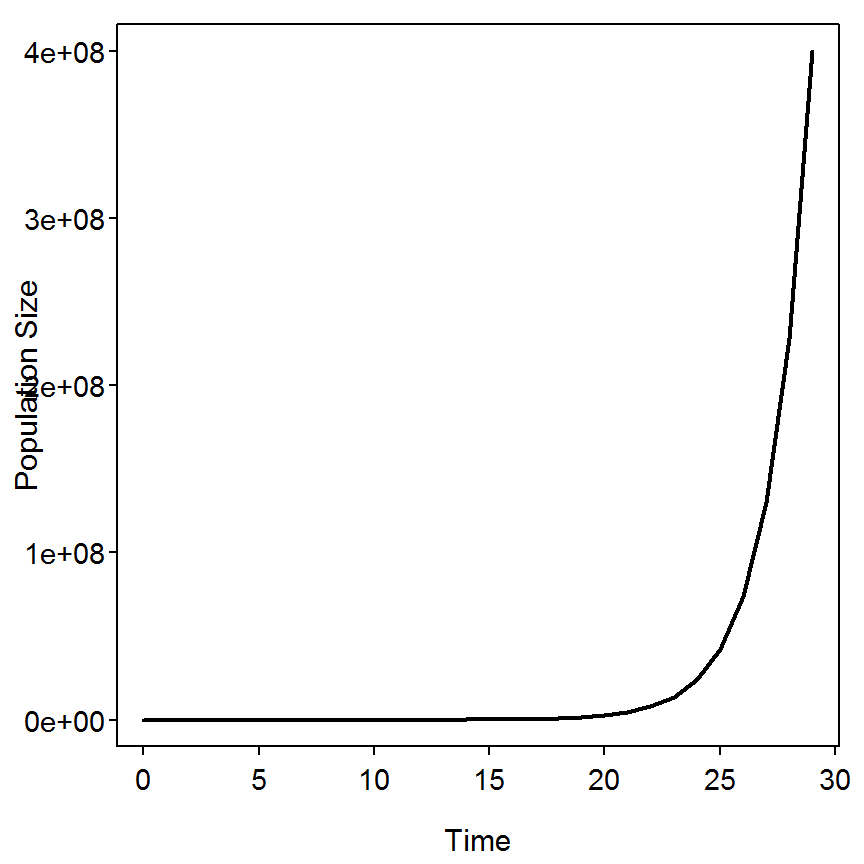The number of animals in each (st)age over time can be seen with stage.vector.plot(). To emphasize the stable age distribution let’s put those results on stage.vector.plot() with abline() using the “horizontal” option (h=). > stage.vector.plot(exp1$stage.vector)
> abline(h=stable.stage(M),lwd=2,lty=3,col="red")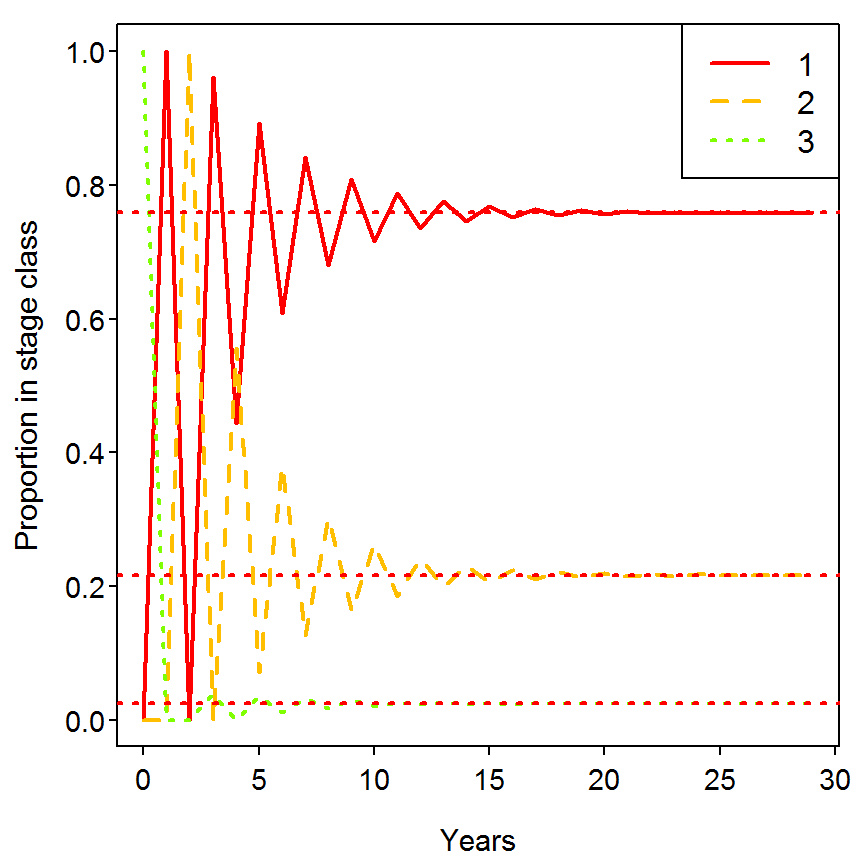### 13.2.4 Modeling Parameter Changes I

In the box, the authors illustrate how to model different scenarios using the Leslie matrix. In their first example, they consider the situation where some pollutant has reduced the survival rate of age-0 fish from 0.5 to 0.4. The corresponding Leslie matrix is entered and the resulting $$\lambda$$ and stable age distribution are found. From this one can see that the annual rate of change decreased from 1.752 to 1.583.

> ( M2 <- matrix(c(0,5,10,0.4,0,0,0,0.2,0),nrow=3,byrow=TRUE) )
     [,1] [,2] [,3]
[1,]  0.0  5.0   10
[2,]  0.4  0.0    0
[3,]  0.0  0.2    0
> M2.eig <- eigen.analysis(M2)
> M2.eig$lambda1  1.582851 > M2.eig$stable.stage
 0.77842857 0.19671557 0.02485586

In their second example, the considered the situation where stocking added 10 more age-1 and 2 more age-2 fish at each time step. Thus, one can see that the annual rate of change decreased from 1.752 to 2.815.

> ( M3 <- matrix(c(0,15,12,0.5,0,0,0,0.2,0),nrow=3,byrow=TRUE) )
     [,1] [,2] [,3]
[1,]  0.0 15.0   12
[2,]  0.5  0.0    0
[3,]  0.0  0.2    0
> M3.eig <- eigen.analysis(M3)
> M3.eig$lambda1  2.815357 > M3.eig$stable.stage
 0.84018524 0.14921470 0.01060006

A graphic presentation of the impact of these changes can be seen by plotting population abundance versus time (left below) or log population abundance versus time (right below) for several time steps. In the code below, note the use of add=TRUE to add the line to an already existing plot and use.log=TRUE to plot log population sizes on the y-axis.

> popSizesPlot(exp1)                                            # base case
> expM2 <- pop.projection(M2,n0,30)
> expM3 <- pop.projection(M3,n0,30)
> legend("topleft",legend=c("base","pollution","stocking"),col=c("black","red","blue"),lwd=2)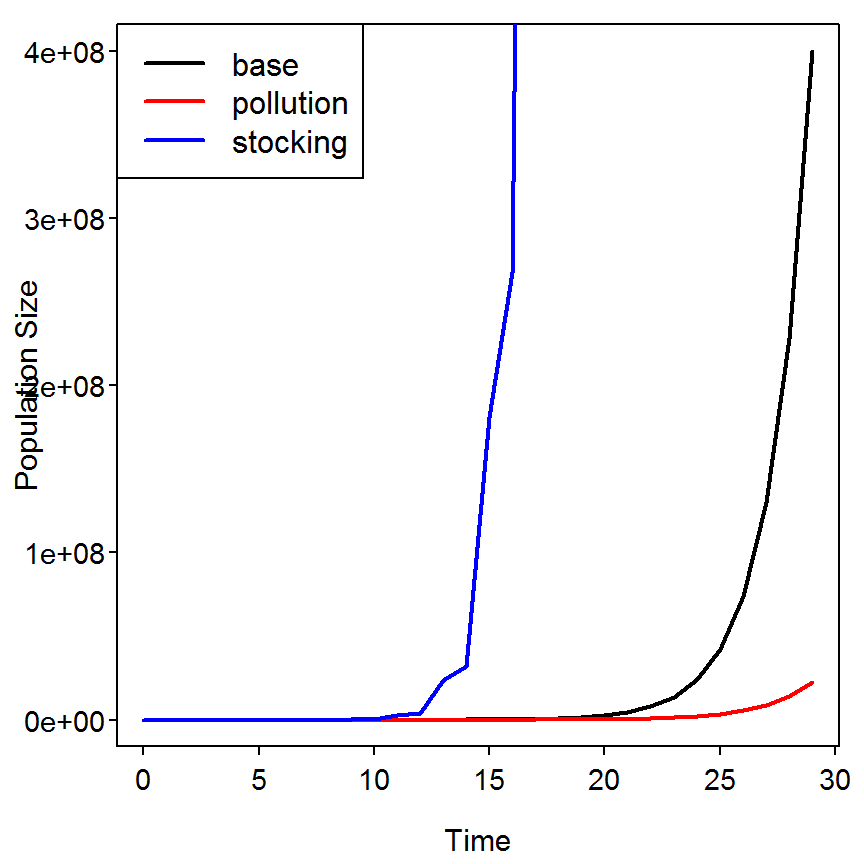> popSizesPlot(exp1,use.log=TRUE)
> popSizesPlot(expM3,add=TRUE,use.log=TRUE,col="blue")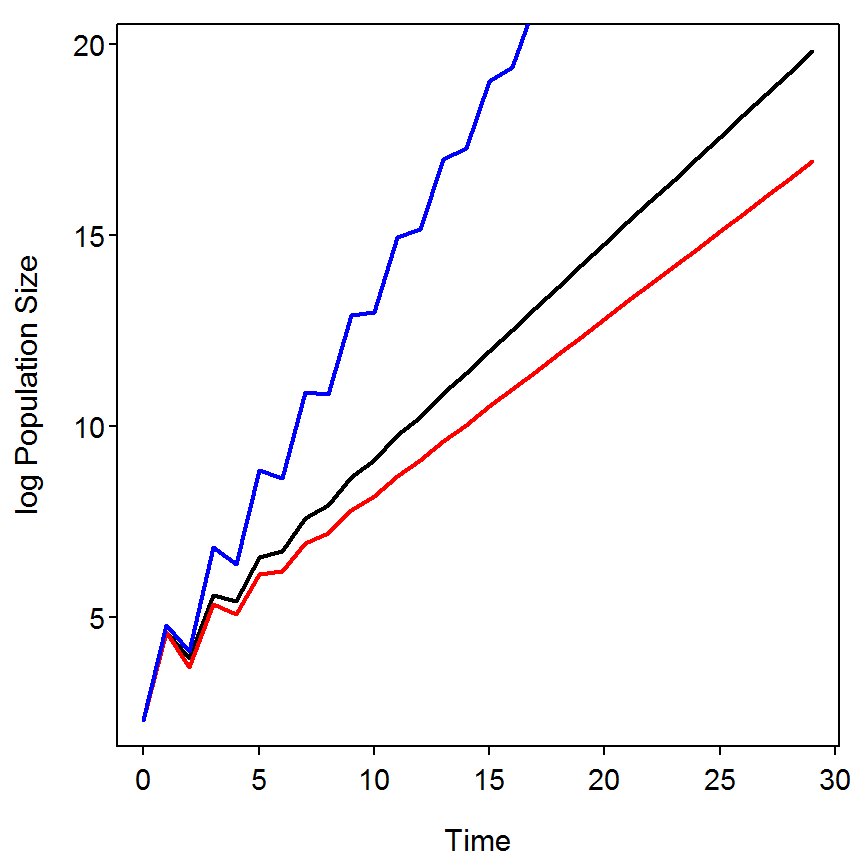### 13.2.5 Modeling Parameter Changes II

In their last example the authors of the box model the rate of population increase ($$r=log(\lambda)$$) against varying values of survival of age-1 fish to model what effect harvest regulations to reduce mortality of age-1 fish would have on overall population growth. It appears that the authors modeled age-1 survival from 0.2 to 0.5 in increments of 0.02. These values can be placed into a vector below. These values of age-1 survival are sequentially placed into the 3rd row and 2nd column of the Leslie matrix to determine the impact on $$r$$. These sequential calculations are most easily accomplished with a loop as shown below.

> ( survs <- seq(0.2,0.5,0.02) )
  0.20 0.22 0.24 0.26 0.28 0.30 0.32 0.34 0.36 0.38 0.40 0.42 0.44 0.46 0.48
 0.50
> iters <- length(survs)                         # get the number of iterations
> rs <- numeric(iters)                           # initiate vector to hold r values
> for (i in 1:iters) {                           # loop i from 1 to number of iterations
Mtemp <- M                                   # put M matrix into Mtemp
Mtemp[3,2] <- survs[i]                       # replace with current survival rate
rs[i] <- log(eigen.analysis(Mtemp)$lambda1) # find r and put in vector of rs } > rs # see if vector is filled   0.5609476 0.5693174 0.5774340 0.5853131 0.5929692 0.6004153 0.6076633  0.6147242 0.6216080 0.6283237 0.6348800 0.6412847 0.6475449 0.6536675  0.6596587 0.6655243 The illustrative plot can then be constructed with, > plot(rs~survs,type="b",pch=19,xlab="Age-1 survival probability", ylab="Rate of population increase (r)") > abline(h=log(eigen.analysis(M)$lambda1),lty=2,col="red")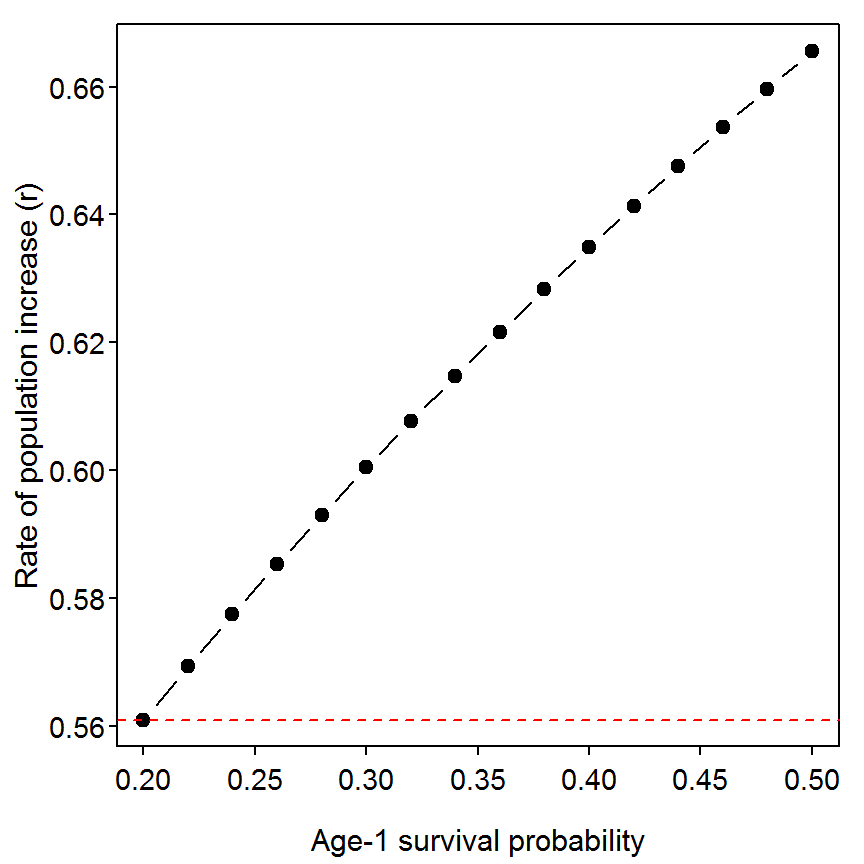## 13.3 Surplus Production Analysis

Consider the data for a commercial Alewife (Alosa pseudoharengus) used in Box 8.7 in the Chapter 8 vignette to illustrate the application of surplus production models.

The parameters initial biomass ($$B_{0}$$), carrying capacity ($$K$$), catchability ($$q$$), and the intrinsic rate of growth ($$r$$) required to solve the model for biomass in period $$t$$ are given as $$B_{0}$$ = 732,506, $$K$$ = 1,160,771; $$q$$ = 0.0001484; and $$r$$ = 0.4049 as determined in the example in Box 8.7. The resulting set of computations form the base case scenario for determining the possible impacts of reductions in the intrinsic rate of population increase (2% decline in $$r$$ to 0.396773), with the latter scenario requiring re-computation of the predicted biomass series by means of equation (13.20). To determine further the offsetting reductions in catch required to compensate for changes in biomass caused by reducing $$r$$, a third set of calculations is performed using equation (13.20) to predict biomass. Example computations are given below for 1.1% and 1.6% reductions in the catch rate.

### 13.3.1 Ogle Comment

I found the following errors/typos in this box.

• The years in the bottom table should be from 1986 through 1990 to match the top table (note that 1989 is missing).
• Reference to equation 13.19 should actually be to equation 13.20.
• The $$r$$ for a 2% decline is 0.396802 rather than 0.396773.
• We are never told what $$p$$ in equation 13.20 is in the box (I assumed $$p=1$$ and it matched the results in the box).
• According to the calculations in this box, the subscript on $$C$$ in equation 13.20 should be $$t+1$$ rather than $$t$$.
• In equation 13.20 there appears to be both a lower-case $$p$$ and an upper-case $$P$$.

### 13.3.2 Computations

The base information or information that does not change among the scenarios was entered to objects.

> year <- c(NA,1986:1990)                        # year labels
> ct <- c(NA,90000,113300,155860,181128,198584)  # catches per year
> iters <- length(year)-1                        # number of iterations required
>
> K <- 1160771                                   # Carrying capacity
> q <- 0.0001484                                 # Catchability coef (given but not used)
> ( B <- c(732506,rep(NA,length(year)-1)) )      # An initialized biomass vector
 732506     NA     NA     NA     NA     NA
> r <- 0.4049                                    # Base intrinsic growth rate

Equation 13.20 was then computed using an iterative processing loop shown in the second line below. First, however, a new vector that will hold the computed biomasses was initialized with B from above.

> B1 <- B
> for (i in 1:iters) B1[i+1] <- B1[i]+r*B1[i]*(1-B1[i]/K)-ct[i+1]
> B1
 732506.0 751933.1 745866.9 697953.8 629503.4 547576.9

In the first change scenario, the intrinsic growth rate was reduced by 2% and the process used to develop the base scenario was repeated.

> ( r <- 0.4049*0.98 )
 0.396802
> B2 <- B
> for (i in 1:iters) B2[i+1] <- B2[i]+r*B2[i]*(1-B2[i]/K)-ct[i+1]

In the second change scenario, the new r was still used, the catch vector was reduced by 1.6%, and the process was repeated.

> ct1 <- ct*0.984
> B3 <- B
> for (i in 1:iters) B3[i+1] <- B3[i]+r*B3[i]*(1-B3[i]/K)-ct1[i+1]

The third change scenario was exactly as above but with a 1.1% change in the catch vector.

> ct2 <- ct*0.989
> B4 <- B
> for (i in 1:iters) B4[i+1] <- B4[i]+r*B4[i]*(1-B4[i]/K)-ct2[i+1]

The results from the base scenario and all three change scenarios are column bound together (with cbind()) for ease of display.

> res <- round(cbind(year,ct,B1,B2,B3,B4),0)  # round for display only
> print(res,na.print="")                      # don't shown NAs
     year     ct     B1     B2     B3     B4
[1,]             732506 732506 732506 732506
[2,] 1986  90000 751933 749745 751185 750735
[3,] 1987 113300 745867 741789 744874 743910
[4,] 1988 155860 697954 692172 697408 695773
[5,] 1989 181128 629503 621922 629646 627235
[6,] 1990 198584 547577 537897 548559 545234

## 13.4 Stock-Recruitment Analysis

The Ricker stock-recruitment curve is used below on hypothetical data (shown in table in box) for a longitudinal study of a stream-dwelling population of Brown Trout (Salmo trutta).

I found the following errors/typos in this box:

• Reference to equations 13.28 and 13.26 on page 598 should both refer to equation 13.30.
• It should be made clear that the equilibrium value is found by dividing log(alpha) by beta and not by logging alpha divided by beta.

### 13.4.2 Preparing Data

The Box13_4.txt data file is read and observed below. In addition, a natural log version of the number of recruits was computed and added as a new variable (logR) to the data frame.

> d4 <- read.table("data/Box13_4.txt",header=TRUE)
> str(d4)
'data.frame':   17 obs. of  3 variables:
$yearclass: int 1985 1986 1987 1988 1989 1990 1991 1992 1993 1994 ...$ stock    : int  1034 505 390 574 1032 642 803 1768 1630 941 ...
$recruits : int 1443 1705 1680 1918 1283 1830 1718 550 758 1475 ... > d4$logR <- log(d4$recruits) } ### Fitting Ricker Stock-Recruitment Model The fitting of stock-recruitment models was described in the Box 4.8 in the Chapter 4 vignette and, in greater detail here. Some of the simplifying functions described there will be used in this vignette. The Ricker stock-recruitment model is declared, starting values are determined, the model is fit to these data, and the model coefficients are extracted as shown below. Thus, the fitted Ricker model has an alpha of 9.1823 and a beta of 0.00185. > sr1 <- srFuns("Ricker",param=1) > ( sr1s <- srStarts(recruits~stock,data=d4,type="Ricker",param=1) ) $a
 9.182347

$b  0.001845013 > sr1r <- nls(logR~log(sr1(stock,a,b)),data=d4,start=sr1s) > coef(sr1r)  a b 9.182347434 0.001845013  The fitted line plot with the 1:1 replacement line for these data is constructed below. This plot illustrates the equilibrium replacement value of 1202. > plot(recruits~stock,data=d4,pch=19,xlim=c(0,2000),ylim=c(0,2000)) > curve(sr1(x,coef(sr1r),coef(sr1r)),from=0,to=2000,col="red",lwd=2,add=TRUE) > abline(coef=c(0,1),col="blue",lwd=2,lty=2)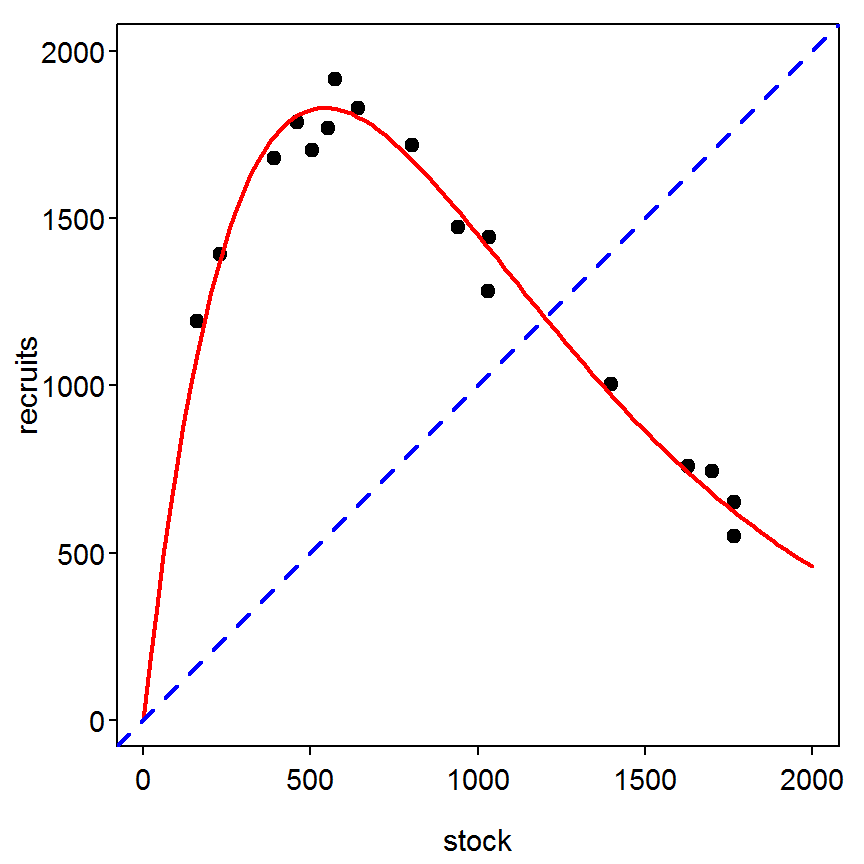As alpha is used extensively in the next section, I will save it to an object for simplicity. Note that this saves many more decimal places then what is used in print in the book; thus, the results below will differ somewhat from the results printed in the book. I also saved beta for the plotting at the end. > ( alpha <- coef(sr1r) )  a 9.182347  > ( beta <- coef(sr1r) )  b 0.001845013  ### 13.4.3 Modeling with Stock-Recruitment Curve In this example, the author supposes that the average adult fecundity ($$E$$) is 1000 and then uses equation 13.30 to determine the population independent mortality rate ($$Z_{i}$$). > E <- 1000 > ( Zi <- log(E) - log(alpha) )  a 4.690472  The author then asks us to consider a situation where the population independent mortality rate increases by 10%. The rearrangement of equation 13.30 allows one to estimate a value of $$\alpha$$ given this new value of $$Z_{i}$$. > ( Zi.new <- Zi*1.10 )  a 5.15952  > ( alpha.new <- E*exp(-Zi.new) )  a 5.744458  Under this scenario the base (shown in red) and new (shown in blue) Ricker stock-recruitment curves are visualized as shown below. From this, one can see that the equilibrium replacement value declined from 1202 to 948. > plot(recruits~stock,data=d4,pch=19,xlim=c(0,2000),ylim=c(0,2000)) > curve(sr1(x,alpha,beta),from=0,to=2000,col="red",lwd=2,add=TRUE) > abline(coef=c(0,1),col="blue",lty=2,lwd=2) > curve(sr1(x,alpha.new,beta),from=0,to=2000,col="blue",lwd=2,add=TRUE)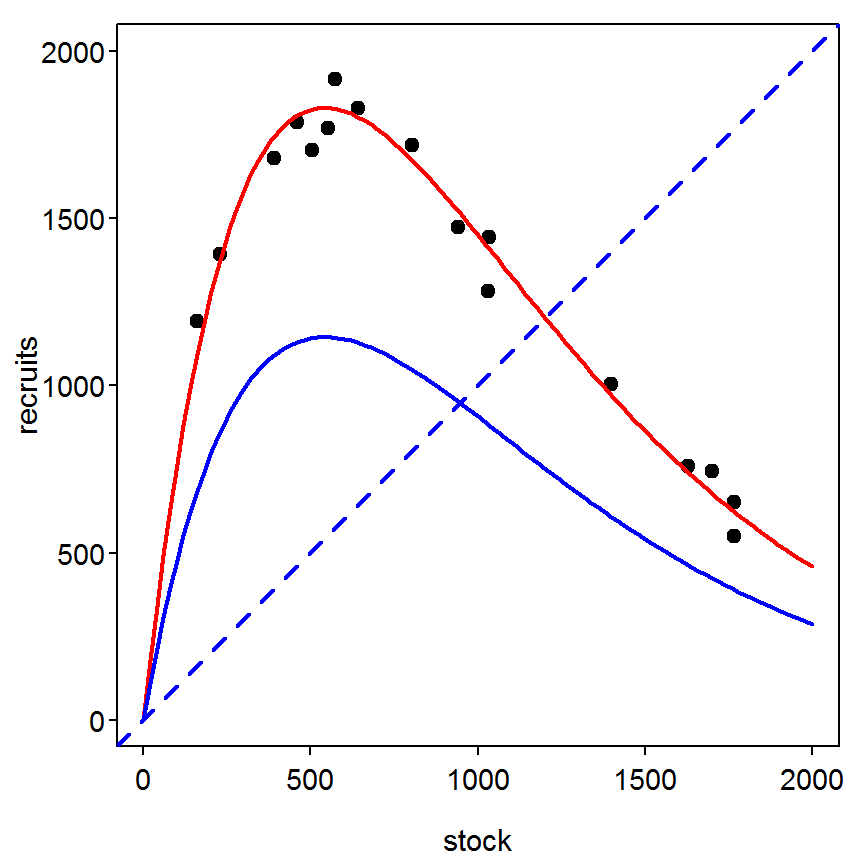The plot on the left below shows the difference in “recruits” between the two lines (negative numbers represent the number of recruits lost by increased population independent mortality) and the difference in recruits as a percentage of the base number of recruits, > stocksize <- 0:max(d4$stock)                 # create stock sizes to model over
> recruits1 <- sr1(stocksize,alpha,beta)       # predict recruits using base model
> recruits2 <- sr1(stocksize,alpha.new,beta)   # predict recruits with increased mortality
> diffrecruits <- recruits2-recruits1          # find difference in predicted recruits
> pdiffrecruits <- diffrecruits/recruits1*100  # express difference as a percentage of base
> plot(diffrecruits~stocksize,type="l",lwd=2,xlab="Stock Size",ylab="Loss of Recruits")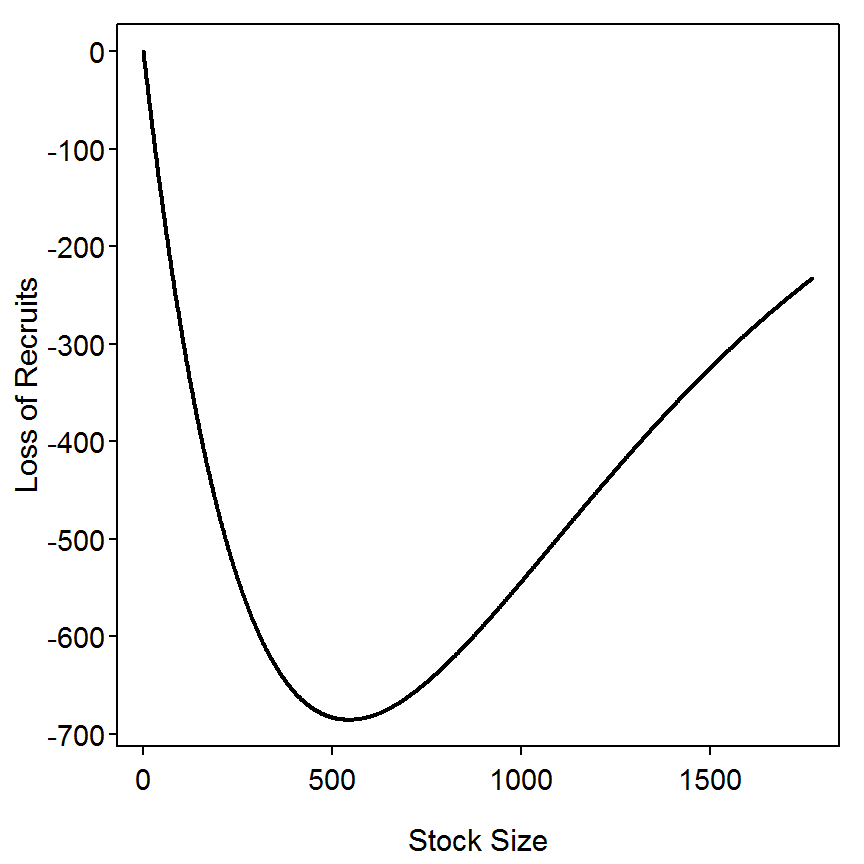> plot(pdiffrecruits~stocksize,type="l",lwd=2,xlab="Stock Size",ylab="Percentage Loss of Recruits")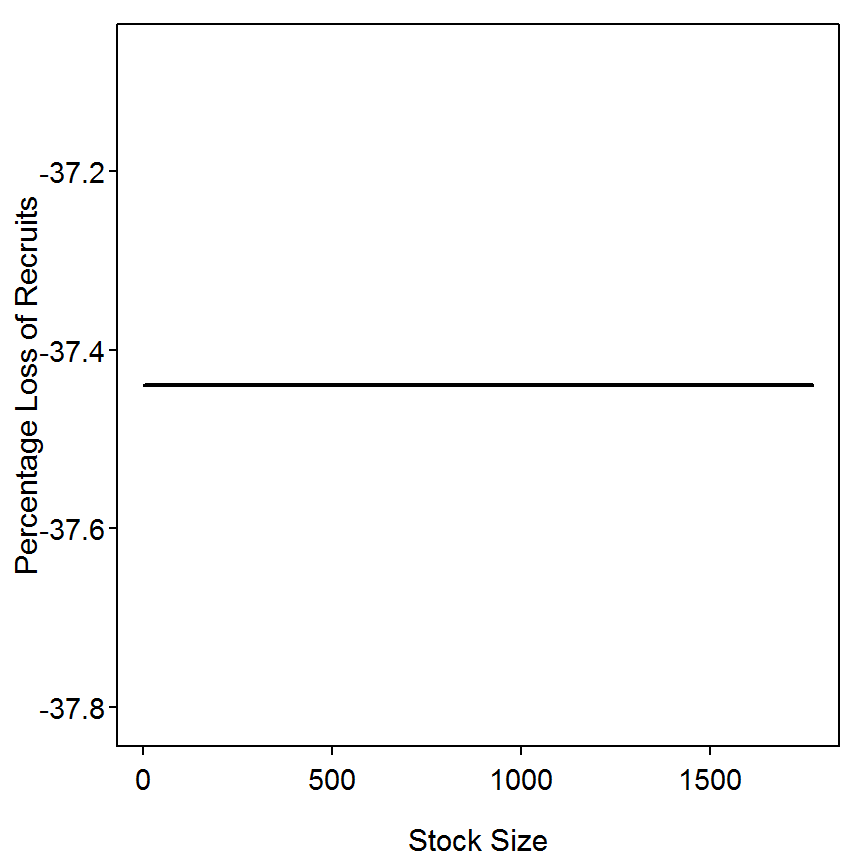## 13.5 Key-Factor Analysis

Consider the data used in Box 13.1 for the 1954 cohort of Brook Trout (Salvelinus fontinalis) from Hunt Creek, Michigan. Those data were expanded to include the 1952 and 1953 cohorts (data shown in table in the box; McFadden et al. (1967)).

### 13.5.1 Ogle Comment

I found the following errors/typos in this box:

• Reference to all equations are off by 1 – e.g., reference to 13.52 should be to 13.53.
• $$K$$ is defined in two different ways on page 607. First it is defined as the ratio of $$N_{m}$$ to $$N_{1}$$ (equation 13.54) and then it is defined as the common log of this ratio (comparison of equations 13.56 and 13.55 and in the sentence just below equation 13.56). In the box, $$K$$ is used as the log of the ratio.
• Major column labels in the last table are backwards. The subtable on the left should be labelled as $$N_{t+1}$$ versus $$N_{t}$$ and the subtable on the right as $$N_{t}$$ versus $$N_{t+1}$$
• y-axis of first figure is labeled with an upper- rather than lower-case $$k$$.
• As a suggestion, I would make it clear in equation 13.55 that COMMON logs are being used (could emphasize this in the lead-in sentence).

### 13.5.2 Preparing Data

The Box13_5.txt data file is read and observed below.

> d5 <- read.table("data/Box13_5.txt",header=TRUE)
> str(d5)
'data.frame':   55 obs. of  3 variables:
$age : int 0 1 2 3 4 0 1 2 3 4 ...$ cohort: int  1949 1949 1949 1949 1949 1950 1950 1950 1950 1950 ...
$abund : int 59500 1970 933 117 7 60000 2144 901 95 6 ... > head(d5,n=10) # first 10 rows  age cohort abund 1 0 1949 59500 2 1 1949 1970 3 2 1949 933 4 3 1949 117 5 4 1949 7 6 0 1950 60000 7 1 1950 2144 8 2 1950 901 9 3 1950 95 10 4 1950 6 These data are in so-called stacked or long format where each age within a cohort appears in a separate row. For ease of some analyses below, the data need to be in so-called unstacked or wide format where all information for one cohort appears in one variable or column. The original data frame can be converted to wide format with reshape(). NEED MORE DESCRIPTION HERE. > d5a <- reshape(d5,v.names="abund",timevar="cohort",idvar="age",direction="wide") > str(d5a) 'data.frame': 5 obs. of 12 variables:$ age       : int  0 1 2 3 4
$abund.1949: int 59500 1970 933 117 7$ abund.1950: int  60000 2144 901 95 6
$abund.1951: int 55500 2516 865 131 12$ abund.1952: int  51000 2694 1052 199 26
$abund.1953: int 40000 3162 1160 183 17$ abund.1954: int  52000 2118 779 159 13
$abund.1955: int 73000 2474 911 193 34$ abund.1956: int  67000 3352 1348 302 24
$abund.1957: int 58500 2548 1144 230 12$ abund.1958: int  75500 2019 1088 208 26
$abund.1959: int 100500 2528 968 200 19 - attr(*, "reshapeWide")=List of 5 ..$ v.names: chr "abund"
..$timevar: chr "cohort" ..$ idvar  : chr "age"
..$times : int 1949 1950 1951 1952 1953 1954 1955 1956 1957 1958 ... ..$ varying: chr [1, 1:11] "abund.1949" "abund.1950" "abund.1951" "abund.19"..

In this format, the results for one cohort can be easily found by using subscripts. For example, the abundance for the 1953 cohort is,

> d5a[,3]             # ask for 3rd column, or
 60000  2144   901    95     6
> d5a[,"abund.1953"]  # by the name of the varible
 40000  3162  1160   183    17
> head(d5a)           # first 10 rows
  age abund.1949 abund.1950 abund.1951 abund.1952 abund.1953 abund.1954
1   0      59500      60000      55500      51000      40000      52000
2   1       1970       2144       2516       2694       3162       2118
3   2        933        901        865       1052       1160        779
4   3        117         95        131        199        183        159
5   4          7          6         12         26         17         13
abund.1955 abund.1956 abund.1957 abund.1958 abund.1959
1      73000      67000      58500      75500     100500
2       2474       3352       2548       2019       2528
3        911       1348       1144       1088        968
4        193        302        230        208        200
5         34         24         12         26         19

For later use an object that contains the number of ages in the data was created.

> ( m <- dim(d5a) )
 5

### 13.5.3 Example Calculations for the 1953 Cohort

The age specific survival rates ($$w_{j}$$) are found with lagratio(), from the FSA package. This function computes the ratio of items in the vector given as the first argument when lagged by one position. In other words, given a vector of abundances ($$N$$) lagratio() will compute $$\frac{N_{t+1}}{N_{t}}$$. The lagged ratio is computed below and the COMMON log of the lagged ratios with the negative signs removed produce the $$k$$ values.

> ( w.1953 <- lagratio(d5a[,"abund.1953"]) )
 0.07905000 0.36685642 0.15775862 0.09289617
> ( k.1953 <- abs(log10(w.1953)) )
 1.1020981 0.4355039 0.8020069 1.0320022

The $$K$$ is then the sum of the $$k$$ values.

> ( K.1953 <- sum(k.1953) )
 3.371611

### 13.5.4 Calculations for All Cohorts

The calculations for all cohorts can be simplified with a loop. The analysis begins by saving the number of cohorts in the analysis into an object. The code then creates a blank matrix to hold the $$k$$ values, using a loop to fill that vector (basically repeating the code from above for each cohort), and then renaming the row and columns of that matrix.

> ( n.coh <- ncol(d5a)-1 )             # number of columns in d5a, -1 (for age column)
 11
> ( k <- matrix(rep(NA,n.coh*(m-1)),nrow=(m-1)) )             # initialize matrix
     [,1] [,2] [,3] [,4] [,5] [,6] [,7] [,8] [,9] [,10] [,11]
[1,]   NA   NA   NA   NA   NA   NA   NA   NA   NA    NA    NA
[2,]   NA   NA   NA   NA   NA   NA   NA   NA   NA    NA    NA
[3,]   NA   NA   NA   NA   NA   NA   NA   NA   NA    NA    NA
[4,]   NA   NA   NA   NA   NA   NA   NA   NA   NA    NA    NA
> for (i in 1:n.coh) k[,i] <- abs(log10(lagratio(d5a[,i+1]))) # need +1 to ignore age col
> rownames(k) <- c("k0","k1","k2","k3")                       # rename rows and col labels
> colnames(k) <- 1949:1959
> round(k,4)                                                  # rounded for display only
     1949   1950   1951   1952   1953   1954   1955   1956   1957   1958   1959
k0 1.4801 1.4469 1.3436 1.2772 1.1021 1.3901 1.4699 1.3008 1.3610 1.5728 1.5994
k1 0.3246 0.3765 0.4637 0.4084 0.4355 0.4344 0.4339 0.3956 0.3478 0.2685 0.4169
k2 0.9017 0.9770 0.8197 0.7232 0.8020 0.6901 0.6740 0.6497 0.6967 0.7186 0.6848
k3 1.2231 1.1996 1.0381 0.8839 1.0320 1.0875 0.7541 1.0998 1.2825 0.9031 1.0223

### 13.5.5 Regression Analysis of $$k$$ Values

In the box, the author first demonstrates plotting the $$k_{i}$$ and $$K$$ values against cohort year to visually estimate which $$k_{i}$$ most closely tracked the trend in $$K$$ and, thus, contributed 11most" to $$K$$. The author used a plot with two y-axes for this procedure. I’m not a fan of graphs with two y-axes so I will make side-by-side plots with the $$k_{i}$$ versus cohort year on one plot and $$K$$ versus cohort year on the other. Before constructing these plots, the vector of $$K$$ values must be created as the sum of the $$k_{i}$$ values for a given cohort year. In addition, it will be easier to work with the k matrix if it is transposed (using t()) so that a set of $$k_{i}$$ values is in a column. Finally, before plotting, the cohort year numbers are extracted and saved to an object. The plot of $$k_{i}$$ values against cohort year is then accomplished with matplot() which takes the x-axis values as the first argument, a matrix of y-axis values as the second argument, and then the usual optional control arguments. The plot of $$K$$ against cohort year is simply accomplished with the familiar plot().

> ( K <- apply(k,2,sum) )
    1949     1950     1951     1952     1953     1954     1955     1956
3.929419 4.000000 3.665112 3.292597 3.371611 3.602060 3.331844 3.445864
1957     1958     1959
3.687975 3.462974 3.723412 
> ( kt <- t(k) )
           k0        k1        k2        k3
1949 1.480051 0.3245846 0.9016958 1.2230878
1950 1.446926 0.3765000 0.9770012 1.1995724
1951 1.343582 0.4636945 0.8197448 1.0380900
1952 1.277173 0.4083819 0.7231627 0.8838797
1953 1.102098 0.4355039 0.8020069 1.0320022
1954 1.390077 0.4343885 0.6901403 1.0874538
1955 1.469923 0.4338813 0.6739611 0.7540784
1956 1.300771 0.3956141 0.6496829 1.0997957
1957 1.360956 0.3477734 0.6966982 1.2825466
1958 1.572811 0.2685074 0.7185656 0.9030900
1959 1.599389 0.4169017 0.6848454 1.0222764
> yrs <- as.numeric(rownames(kt))
> matplot(yrs,kt,type="l",lwd=2,ylim=c(0,2),xlab="Cohort",ylab="k(i) values")
> legend("topleft",c("k0","k1","k2","k3"),lty=1:4,lwd=2,col=1:4,cex=0.8)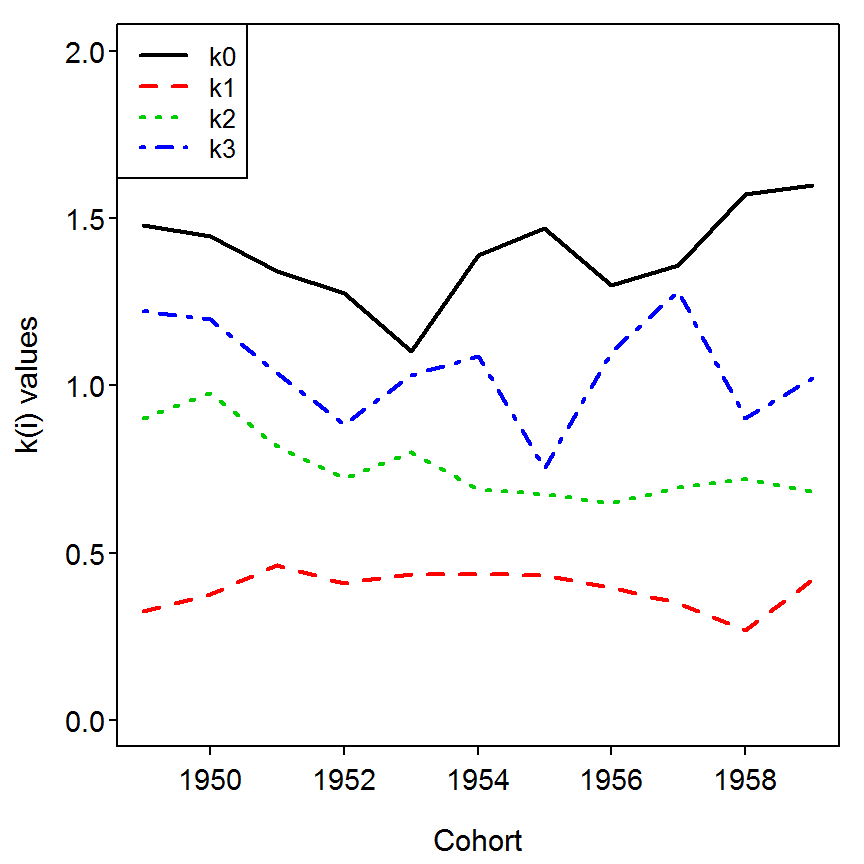> plot(K~yrs,type="l",lwd=2,ylim=c(0,max(K)),xlab="Cohort",ylab="K")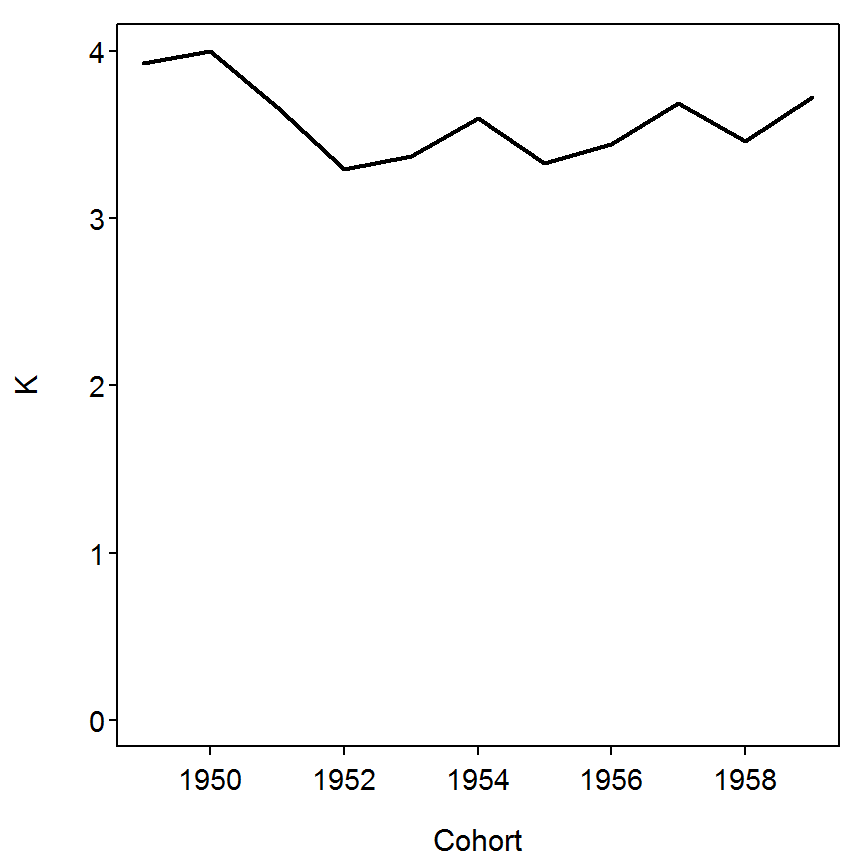The author also provided a table that summarized the slope and r-square values from the regressions of a $$k_{i}$$ on $$K$$. These results, for $$k_{0}$$, are obtained with lm() and by submitting the saved lm object to summary().

> lm1 <- lm(kt[,1]~K)
> summary(lm1)

Call:
lm(formula = kt[, 1] ~ K)

Residuals:
Min       1Q   Median       3Q      Max
-0.23696 -0.05765 -0.04188  0.07034  0.21062

Coefficients:
Estimate Std. Error t value Pr(>|t|)
(Intercept)   0.4853     0.6548   0.741    0.478
K             0.2532     0.1819   1.392    0.197

Residual standard error: 0.1357 on 9 degrees of freedom
Multiple R-squared:  0.1772,    Adjusted R-squared:  0.08573
F-statistic: 1.938 on 1 and 9 DF,  p-value: 0.1974

The following loop is used to compute all four of these regressions, extract the desired results, and provide a summary table.

> slps <- slpp <- rsqs <- numeric(4)             # initialize vector to hold results
> for (i in 1:4) {                               # look through k values
lm1 <- lm(kt[,i]~K)                          # fit regression
slps[i] <- coef(lm1)                      # extract slope
slpp[i] <- anova(lm1)[1,"Pr(>F)"]            # extract slope p-value
rsqs[i] <- cor(kt[,i],K)^2                   # extract r-squared
}
> round(cbind(slps,slpp,rsqs),4)                 # summary table
        slps   slpp   rsqs
[1,]  0.2532 0.1974 0.1772
[2,] -0.0618 0.4551 0.0634
[3,]  0.3051 0.0195 0.4722
[4,]  0.5034 0.0074 0.5678

### 13.5.6 Regression Analysis to Demonstrate Density Dependence

The author also demonstrated a method for determining if there was density dependent mortality demonstrated within any transition between age-classes. This analysis is simplified by first omitting the age column from the data frame of abundance values. Vector of adjacent ages then needs to be extracted from this matrix. The code below extracts the common logarithm of the age-1 data and places it into a vector called Ntp1 and then extracts the same for age-0 data and places it into a vector called Ntp.

> ( d5b <- d5a[,-1]  )                             # delete age column for simplicity
  abund.1949 abund.1950 abund.1951 abund.1952 abund.1953 abund.1954 abund.1955
1      59500      60000      55500      51000      40000      52000      73000
2       1970       2144       2516       2694       3162       2118       2474
3        933        901        865       1052       1160        779        911
4        117         95        131        199        183        159        193
5          7          6         12         26         17         13         34
abund.1956 abund.1957 abund.1958 abund.1959
1      67000      58500      75500     100500
2       3352       2548       2019       2528
3       1348       1144       1088        968
4        302        230        208        200
5         24         12         26         19
> ( Ntp1 <- log10(as.numeric(d5b[2,])) )
  3.294466 3.331225 3.400711 3.430398 3.499962 3.325926 3.393400 3.525304
 3.406199 3.305136 3.402777
> ( Ntp <- log10(as.numeric(d5b[1,])) )
  4.774517 4.778151 4.744293 4.707570 4.602060 4.716003 4.863323 4.826075
 4.767156 4.877947 5.002166

Now the regression of the “earlier age” on the “latter age” is fit with lm() and the coefficients extracted with coef(). As the author of the box describes, the hypothesis test of interest is whether the slope from this regression is different from one or not. The hoCoef() function (from the FSA package) is designed to assist with performing non-default hypothesis tests with regression coefficients. This function requires the fitted model object as the first argument, an integer indicating which coefficient is being tests (i.e., slope=2), and the specific value in the null hypothesis (i.e., testing against a value of 1). The result indicates that the slope is significant different (i.e., lower) than 1 ($$p=0.0180$$).

> res1 <- lm(Ntp~Ntp1)
> coef(res1)
(Intercept)        Ntp1
5.83896    -0.31004 
> hoCoef(res1,2,1)
 term Ho Value Estimate Std. Error         T    p value
2        1 -0.31004  0.4536832 -2.887566 0.01795532

A similar test for the regression in the other direction is performed with,

> res2 <- lm(Ntp1~Ntp)
> coef(res2)
(Intercept)         Ntp
4.1540141  -0.1591107 
> hoCoef(res2,2,1)
 term Ho Value   Estimate Std. Error         T      p value
2        1 -0.1591107  0.2328275 -4.978409 0.0007610939

Finally, similar results for all ages can be obtained through the following loop,

> slps1 <- slpp1 <- t1 <- numeric(4)      # initial vectors for 1st regression results
> slps2 <- slpp2 <- t2 <- numeric(4)      # initial vectors for 2nd regression results
> for (i in 1:4) {                        # loop through ages
tmp2 <- log10(as.numeric(d5b[i+1,]))
tmp1 <- log10(as.numeric(d5b[i,]))
lm1 <- lm(tmp2~tmp1)                  # first regression
slps1[i] <- coef(lm1)              # extract results
temp <- hoCoef(lm1,2,1)
slpp1[i] <- temp[1,"p value"]
t1[i] <- temp[1,"T"]
lm2 <- lm(tmp1~tmp2)                  # second regression
slps2[i] <- coef(lm2)              # extract results
temp <- hoCoef(lm2,2,1)
slpp2[i] <- temp[1,"p value"]
t2[i] <- temp[1,"T"]
}
> round(cbind(slps1,t1,slpp1,slps2,t2,slpp2),4)  # summary table
       slps1      t1  slpp1   slps2      t2  slpp2
[1,] -0.1591 -4.9784 0.0008 -0.3100 -2.8876 0.0180
[2,]  0.6175 -1.7161 0.1203  0.7454 -0.9462 0.3688
[3,]  1.4994  1.0348 0.3278  0.3451 -5.8955 0.0002
[4,]  1.3223  0.9142 0.3844  0.4612 -4.3818 0.0018

### 13.5.7Elliott (1994) Method

At the very end of the box, the author illustrates a method due to Elliott (1994) for demonstrating if density dependent mortality exists among age classes. These results are demonstrated for age-0 fish below.

> tmpN <- as.numeric(d5b[1,])                    # isolate abundance data for age-0
> tmpK <- as.numeric(k[1,])                      # isolate k data for age-0
> lm1 <- lm(tmpK~tmpN)                           # regression fitting
> summary(lm1)                                   # extract results

Call:
lm(formula = tmpK ~ tmpN)

Residuals:
Min        1Q    Median        3Q       Max
-0.127123 -0.048953  0.002496  0.073807  0.110096

Coefficients:
Estimate Std. Error t value Pr(>|t|)
(Intercept) 9.405e-01  1.106e-01   8.503 1.36e-05
tmpN        7.217e-06  1.707e-06   4.227  0.00222

Residual standard error: 0.08656 on 9 degrees of freedom
Multiple R-squared:  0.665, Adjusted R-squared:  0.6278
F-statistic: 17.87 on 1 and 9 DF,  p-value: 0.002217

A plot showing the model fit can be obtained with fitPlot(), from the FSA package.

> fitPlot(lm1,xlab="Initial Age-0 Density",ylab="k-value from Age-0 to Age-1",main="")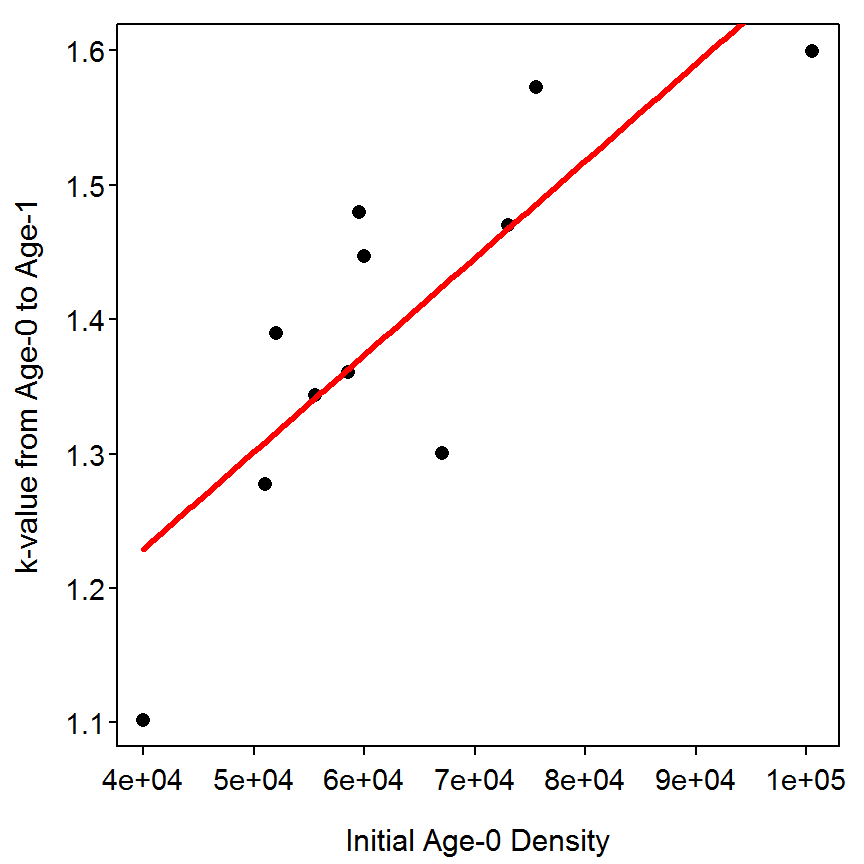Similar results for all age-classes can be obtained with the following loop.

> slpsE <- slppE <- tE <- numeric(4)             # initialize results vectors
> for (i in 1:4) {                               # loop through ages
tmpN <- as.numeric(d5b[i,])
tmpK <- as.numeric(k[i,])
lm1 <- lm(tmpK~tmpN)                         # fit regression
slpsE[i] <- coef(lm1)                     # extract results
temp <- hoCoef(lm1,2)
slppE[i] <- temp[1,"p value"]
tE[i] <- temp[1,"T"]
}
> round(cbind(slpsE,tE,slppE),5)                 # summary table
        slpsE       tE   slppE
[1,]  0.00001  4.22680 0.00222
[2,]  0.00006  1.54454 0.15686
[3,] -0.00022 -1.11647 0.29314
[4,] -0.00054 -0.60029 0.56312

Reproducibility Information
Compiled Date: Fri May 15 2015
Compiled Time: 6:23:11 PM
Code Execution Time: 4.91 s

R Version: R version 3.2.0 (2015-04-16)
System: Windows, i386-w64-mingw32/i386 (32-bit)
Base Packages: base, datasets, graphics, grDevices, methods, stats, utils
Required Packages: FSA, TTR, popbio and their dependencies (car, dplyr,
gdata, gplots, Hmisc, knitr, lmtest, multcomp, plotrix, quadprog,
relax, sciplot, xts)
Other Packages: car_2.0-25, FSA_0.6.13, knitr_1.10.5, plyr_1.8.2,
zoo_1.7-12
caTools_1.17.1, cluster_2.0.1, codetools_0.2-11, colorspace_1.2-6,
DBI_0.3.1, digest_0.6.8, dplyr_0.4.1, evaluate_0.7, foreign_0.8-63,
formatR_1.2, Formula_1.2-1, gdata_2.16.1, ggplot2_1.0.1, gplots_2.17.0,
grid_3.2.0, gridExtra_0.9.1, gtable_0.1.2, gtools_3.4.2, highr_0.5,
Hmisc_3.16-0, htmltools_0.2.6, KernSmooth_2.23-14, lattice_0.20-31,
latticeExtra_0.6-26, lme4_1.1-7, lmtest_0.9-33, magrittr_1.5,
MASS_7.3-40, Matrix_1.2-0, mgcv_1.8-6, minqa_1.2.4, multcomp_1.4-0,
munsell_0.4.2, mvtnorm_1.0-2, nlme_3.1-120, nloptr_1.0.4, nnet_7.3-9,
parallel_3.2.0, pbkrtest_0.4-2, plotrix_3.5-11, proto_0.3-10,
quantreg_5.11, RColorBrewer_1.1-2, Rcpp_0.11.6, relax_1.3.15,
reshape2_1.4.1, rpart_4.1-9, sandwich_2.3-3, scales_0.2.4,
sciplot_1.1-0, SparseM_1.6, splines_3.2.0, stringi_0.4-1,
stringr_1.0.0, survival_2.38-1, TH.data_1.0-6, tools_3.2.0, yaml_2.1.13

## References

Elliott, J. M. 1994. Quantitative ecology and the brown trout. Oxford University Press, Oxford, UK.

McFadden, J. T., G. R. Alexander, and D. S. Shetter. 1967. Numerical changes and population regulation in brook trout Salvelinus fontinalis. Journal of the Fisheries Research Board of Canada 24:1425–1459.